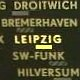# Jacobian Matrix

casio fx-9860GII programming numerical methods derivatives

4 replies to this topic

### #1TOM_DLB_89

TOM_DLB_89

Newbie

•• Members
•• 4 posts
• Gender:Not Telling

• Calculators:
Casio fx-9860 GII
Casio fx-991ES PLUS
Casio fx-82ES PLUS

Posted 15 February 2018 - 09:36 AM

I've made a program that solves non-linear systems (2x2 and 3x3) of equations by newton method: r[i+1]=r[i]-J(r[i])^-1*F(r[i]).

The user is able to insert the functions (that I've set the program to store in FMEM - fn1, fn2...). During the iteration the values of each function will be in the vector F.

The big deal is defining the Jacobian Matrix. I wasn't able to find any way to make it unless the user insert by itself all the derivatives (4 or 9 functions) and the program allocate them, after calculating its values, in the matrix J.

Any suggestions or tips?

### #2pan.gejt

pan.gejt

Casio Freak

•• Members
•• 247 posts
• Gender:Male
• Location:CZ

• Calculators:
.

Posted 15 February 2018 - 06:23 PM

Since there is no CAS inside 9860G II you have to calculate the derivatives numerically. Unfortunatelly, the numerical differentiation built in the calc is for single variable functions only, e.g. f(x)=x^3-ln(x)+ x.

I don't know how to calculate the derivative numerically for function with two or more variables, e.g. f(x)=x^2*y.

The syntax is d/dx(fx),a,tol)

### #3piu58

piu58

Casio Freak

•• Members
•• 142 posts
• Gender:Male

• Calculators:
Casio fx-9860G, Casio fx-CG20

Posted 15 February 2018 - 07:53 PM

It is not difficult to differentiate numerical. You need to define your function as a subroutine. To get the derivate at a point x, simply calc f(x) and f(x+d), where d is a small (but not too small) difference of the original x. The derivate ist the difference of the values divided by d.

### #4TOM_DLB_89

TOM_DLB_89

Newbie

•• Members
•• 4 posts
• Gender:Not Telling

• Calculators:
Casio fx-9860 GII
Casio fx-991ES PLUS
Casio fx-82ES PLUS

Posted 15 February 2018 - 07:59 PM

Yes, that is my problem. I need to input manually the partial derivatives formulas so the program will calculate the value.
Most of the times I get some equations like thoseand it is a hard work writing down the derivatives hahahaha.

Is there any way to solve non linear systems in casio fx-9860GII? I mean, systems like the one above. (I know that some systems are 'solvable' with the intersect function in graph mode).

*edit: K1, K2, na, nb, nc, nd, and ne are constants. The variables are E1 and E2.

Edited by TOM_DLB_89, 15 February 2018 - 08:01 PM.

### #5slugrustle

slugrustle

Newbie

•• Members
•• 8 posts
• Gender:Not Telling

• Calculators:
fx-5800P
fx-9860GII SD

Posted 24 November 2018 - 06:21 PM

piu58 has it right, although I would use the central or symmetric finite difference instead of the forward finite difference.

Given a two-variable function f(x,y), ∂f/∂x ≈ (f(x+h,y) - f(x-h,y)) ÷ (2×h), and ∂f/∂y ≈ (f(x,y+h) - f(x,y-h)) ÷ (2×h). h should be small relative to the variable against which you are differentiating, but not so small that you run into truncation error during subtraction to form the numerator. This can be extended to any number of function arguments. You can see these equations printed nicely at https://en.wikipedia...veral_variables

If accuracy becomes an issue with finite differences, you can use Richardson Extrapolation to improve the accuracy.

If you have the time and the interest, I recommend using either the Levenberg–Marquardt algorithm or Trond Steihuag's Conjugated Gradient method (http://www.ii.uib.no...apers/trust.pdf) and solving the update step with a QR decomposition instead of using a straight inverse or applying Cholesky Decomposition to the normal equations. I have had great success with both of these in my previous job, which largely involved programming and optimizing nonlinear solvers.

### Also tagged with one or more of these keywords: casio fx-9860GII, programming, numerical methods, derivatives

#### 0 user(s) are reading this topic

0 members, 0 guests, 0 anonymous users